# Math

 Results: Mastery of Math Facts Efficient: 5 Minutes per Day Versatile: Individual or Small Groups Evidence Based: Precision Teaching Methods Comprehension: Allows Creative Insight

The Oral Calculation Series uses flip charts in an easel format designed to provide daily oral practice mastering math facts. The program can be used with an entire classroom, in a learning center or for individualized tutoring. Students accomplish a great deal of daily work with instant feedback. The instructor immediately corrects mistakes and then the student writes the correct mathematical fact. This multi-sensory approach very efficiently leads students to mastery of math facts.

These books have been designed as a standalone easel so that one student sees the problems and the instructor or another student sees the problems with the highlighted answers. The student works on daily one-minute exercises in math fluency.

The individual books include numeration, addition, subtraction, multiplication, division, fractions, and decimals.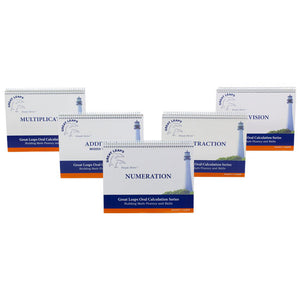BASIC ORAL CALCULATION PACKAGE

The Basic Calculation Set includes Numeration, Addition and Mixed Facts, Subtraction, Multiplication and Division. Our math programs are a simple, multi-sensory approach to teaching basic math facts.

FOR STUDENTS: All ages who have not mastered the basic math facts

INSTRUCTION: One instructor or peer with one student or one...

Regular price \$125.00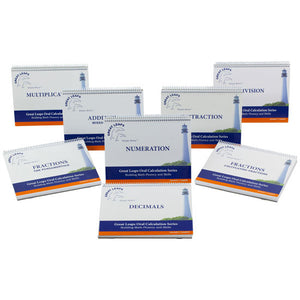COMPLETE ORAL CALCULATION PACKAGE

The Complete Oral Calculation Set includes Numeration, Addition and Mixed Facts, Subtraction, Multiplication, Division, Fraction Fundamentals, Working with Fractions and Decimals. Our math programs are a simple, multi-sensory approach to teaching basic math facts.

FOR STUDENTS: All ages who have not mastered the basic math facts

INSTRUCTION: One instructor...

Regular price \$200.00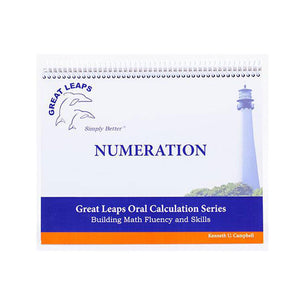ORAL CALCULATION - NUMERATION

Designed to teach children to recognize their numbers and understand the value given to a written number. Our math programs are a simple, multi-sensory approach to teaching basic math facts.

FOR STUDENTS: All ages who have not mastered addition INSTRUCTION: One instructor or peer with one student or one...

Regular price \$25.00ORAL CALCULATION - ADDITION MIXED FACTS

This program teaches addition facts to 18 to automatically finishing with some work in mixed addition. Our math programs are a simple, multi-sensory approach to teaching basic math facts.

FOR STUDENTS: All ages who have not mastered addition INSTRUCTION: One instructor or peer with one student or one teacher...

Regular price \$30.00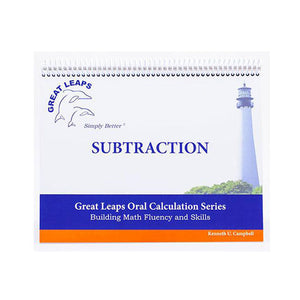ORAL CALCULATION - SUBTRACTION

This program teaches subtraction facts from up to 18 to automatically. Our math programs are a simple, multi-sensory approach to teaching basic math facts.

FOR STUDENTS: All ages who have not mastered subtraction INSTRUCTION: One instructor or peer with one student or one teacher supervising a small group

Regular price \$30.00ORAL CALCULATION - MULTIPLICATION

This program teaches the 0 - 9 tables to automatically. Increased skills in arithmetic lends to faster processing in later, more complex areas of mathematics. Our math programs are a simple, multi-sensory approach to teaching basic math facts.

FOR STUDENTS: All ages who have not mastered multiplication INSTRUCTION: One instructor...

Regular price \$30.00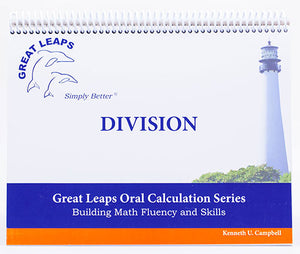ORAL CALCULATION - DIVISION

Teaches students their division facts through the 9’s to proficiency. Our math programs are a simple, multi-sensory approach to teaching basic facts.

FOR STUDENTS: All ages who have not mastered division

INSTRUCTION: One instructor or peer with one student or one teacher supervising a small group

Regular price \$30.00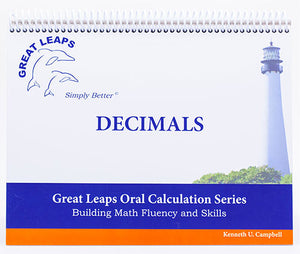ORAL CALCULATION - DECIMALS

This program gives students the ability to recognize decimal places; add and subtract decimals; convert decimals to fractions; then simplify and convert fractions back to decimals or percentages.

FOR STUDENTS: Of all ages who not mastered calculations with decimals
INSTRUCTION: One instructor with one student

Regular price \$40.00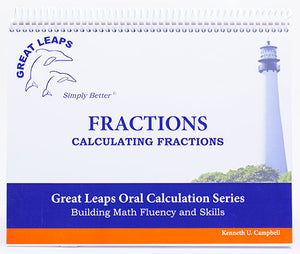ORAL CALCULATION - FRACTIONS CALCULATING FRACTIONS

Calculating fractions utilizes the basic skills that the students have acquired through the previous programs. This program develops their skills in adding, subtracting and simplifying fractions.

FOR STUDENTS: Of all ages who have not mastered the fundamentals of fractions
INSTRUCTION: One instructor with one student

Regular price \$40.00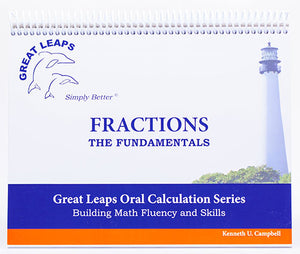ORAL CALCULATION - FRACTIONS THE FUNDAMENTALS

This utilizes the basic skills that the students have acquired through the previous programs. This develops their skills in adding, subtracting and simplifying fractions. Our math programs are a simple, multi-sensory approach to teaching basic math facts.

FOR STUDENTS: Of all ages who have not mastered the fundamentals of fractions...
Regular price \$40.00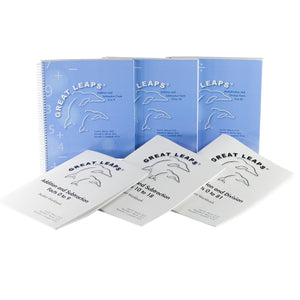Dr. Cecil Mercer's Math Package

This math program was written by Dr. Cecil Mercer for teaching addition, subtraction, multiplication and division. The four components taught are the understanding of math facts and operations, the concepts and rules, progress monitoring with corrective feedback and practice until mastery.

FOR STUDENTS: With significant learning difficulties

INSTRUCTION: One...

Regular price \$200.00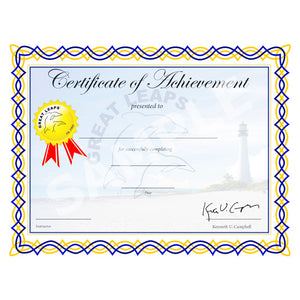ACHIEVEMENT CERTIFICATE

Great Leaps Achievement Certificate.

This is a framable Certificate that rewards students for their accomplishments with tangible recognition they can proudly display.

The certificates are available in packages of 5 or 25.

\$10.00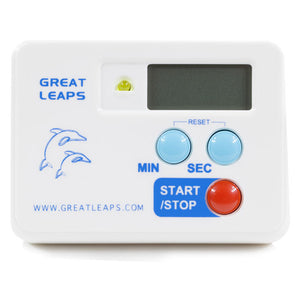DIGITAL COUNTDOWN TIMER

The digital countdown timer accurately measures one exact minute with the choice of either a buzzer or light to indicate when the time is up. For students easily distracted, this light is a must. This versatile timer can lay flat, clip to a book, be used as a magnet, or be...

Regular price \$13.75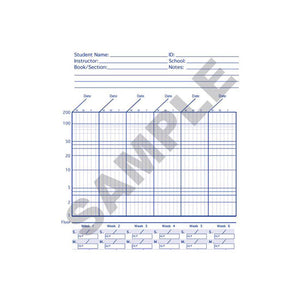PROGRESS CHARTS

Progress Charts
(100 sheet packs)

The proprietary Great Leaps Progress Chart developed from the Standard Behavior Chart by Kenneth Campbell is an equal ratio one-minute chart.  The chart shows relevant movement whereas the usual equal interval chart - or graph paper - cannot.
The Great Leaps Progress Chart shows relevant movement via percentage gains – making it...

Regular price \$15.00